Info

### # 一、动态连通性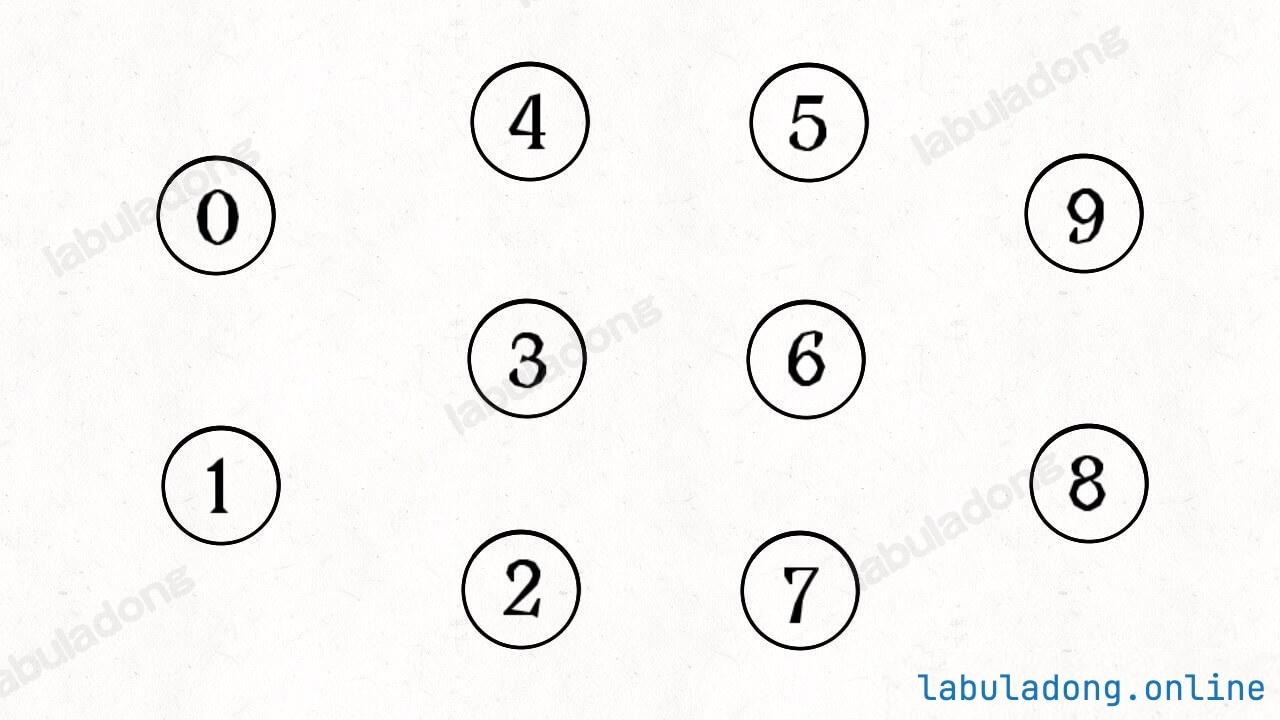``````class UF {
/* 将 p 和 q 连接 */
public void union(int p, int q);
/* 判断 p 和 q 是否连通 */
public boolean connected(int p, int q);
/* 返回图中有多少个连通分量 */
public int count();
}
``````

1、自反性：节点 `p``p` 是连通的。

2、对称性：如果节点 `p``q` 连通，那么 `q``p` 也连通。

3、传递性：如果节点 `p``q` 连通，`q``r` 连通，那么 `p``r` 也连通。### # 二、基本思路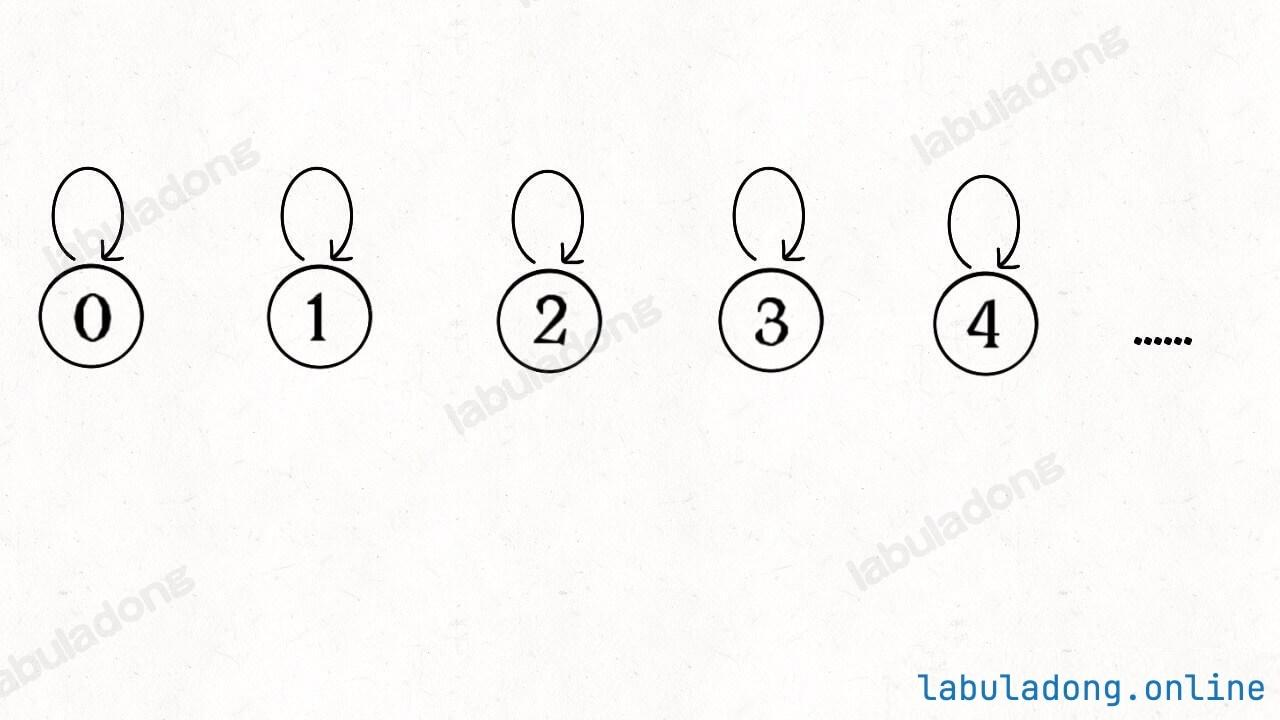``````class UF {
// 记录连通分量
private int count;
// 节点 x 的父节点是 parent[x]
private int[] parent;

/* 构造函数，n 为图的节点总数 */
public UF(int n) {
// 一开始互不连通
this.count = n;
// 父节点指针初始指向自己
parent = new int[n];
for (int i = 0; i < n; i++)
parent[i] = i;
}

/* 其他函数 */
}
``````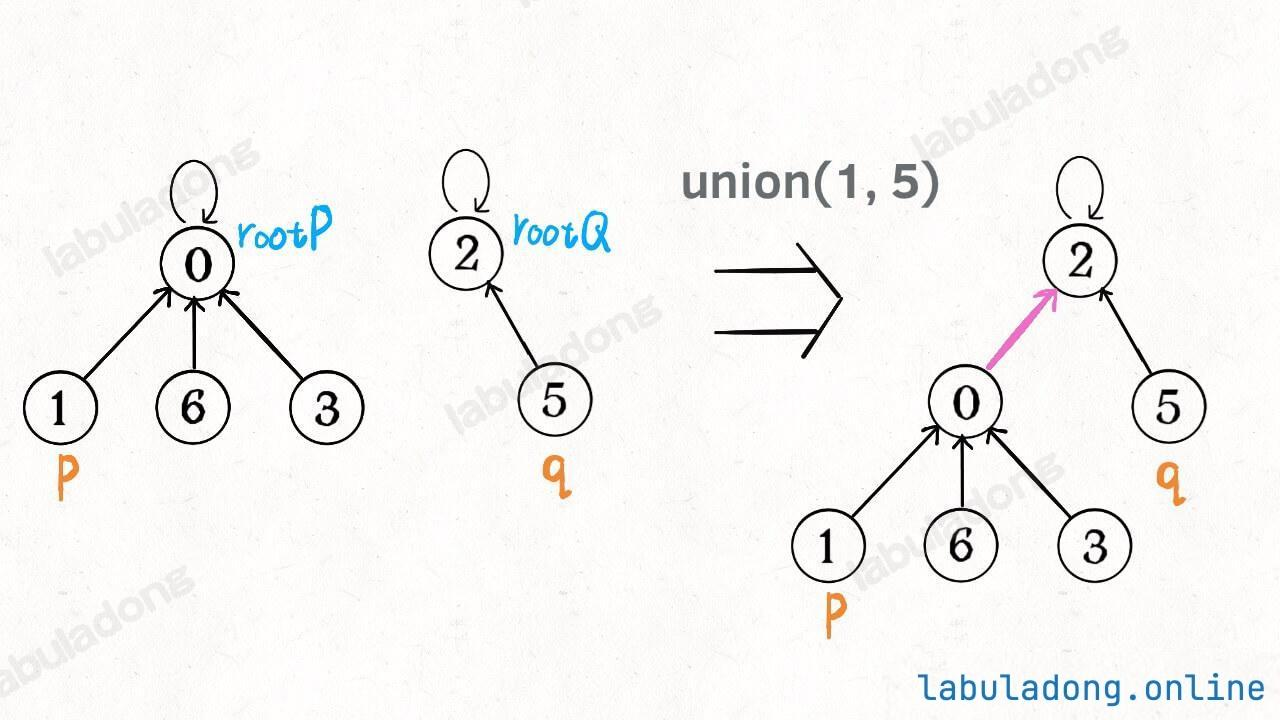``````class UF {
// 为了节约篇幅，省略上文给出的代码部分...

public void union(int p, int q) {
int rootP = find(p);
int rootQ = find(q);
if (rootP == rootQ)
return;
// 将两棵树合并为一棵
parent[rootP] = rootQ;
// parent[rootQ] = rootP 也一样
count--; // 两个分量合二为一
}

/* 返回某个节点 x 的根节点 */
private int find(int x) {
// 根节点的 parent[x] == x
while (parent[x] != x)
x = parent[x];
return x;
}

/* 返回当前的连通分量个数 */
public int count() {
return count;
}
}
``````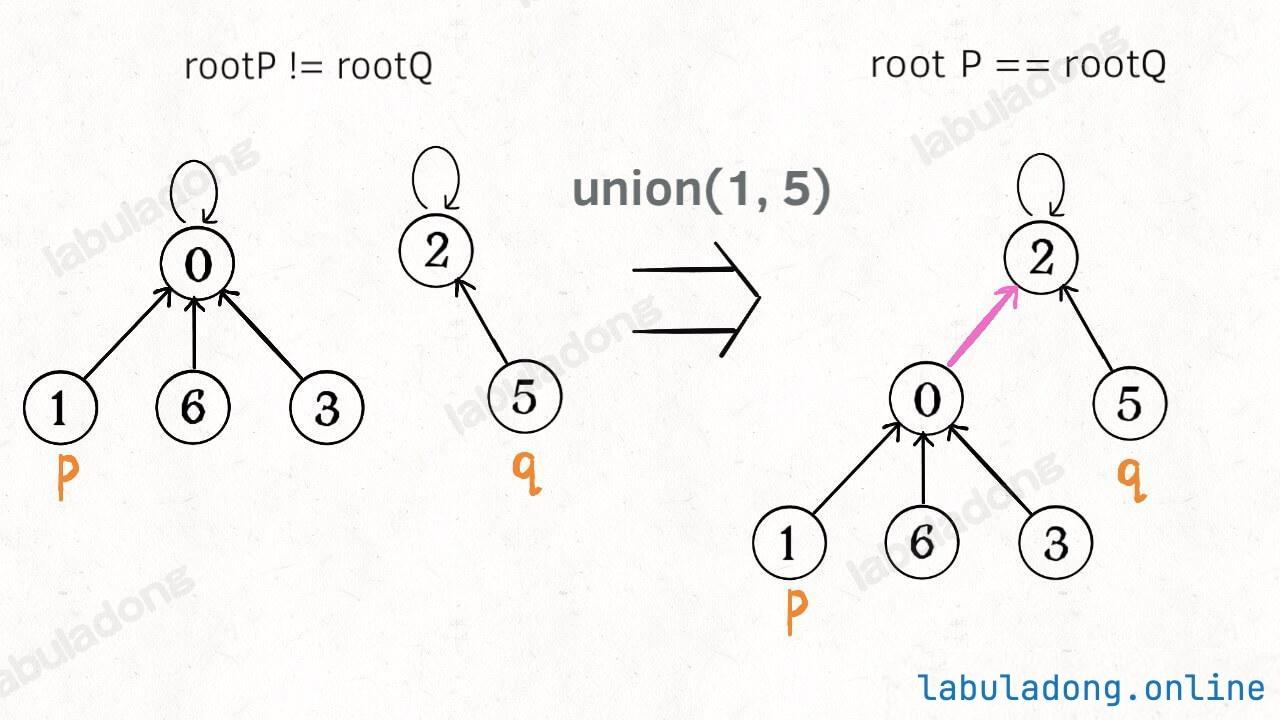``````class UF {
// 为了节约篇幅，省略上文给出的代码部分...

public boolean connected(int p, int q) {
int rootP = find(p);
int rootQ = find(q);
return rootP == rootQ;
}
}
``````

`find` 主要功能就是从某个节点向上遍历到树根，其时间复杂度就是树的高度。我们可能习惯性地认为树的高度就是 `logN`，但这并不一定。`logN` 的高度只存在于平衡二叉树，对于一般的树可能出现极端不平衡的情况，使得「树」几乎退化成「链表」，树的高度最坏情况下可能变成 `N`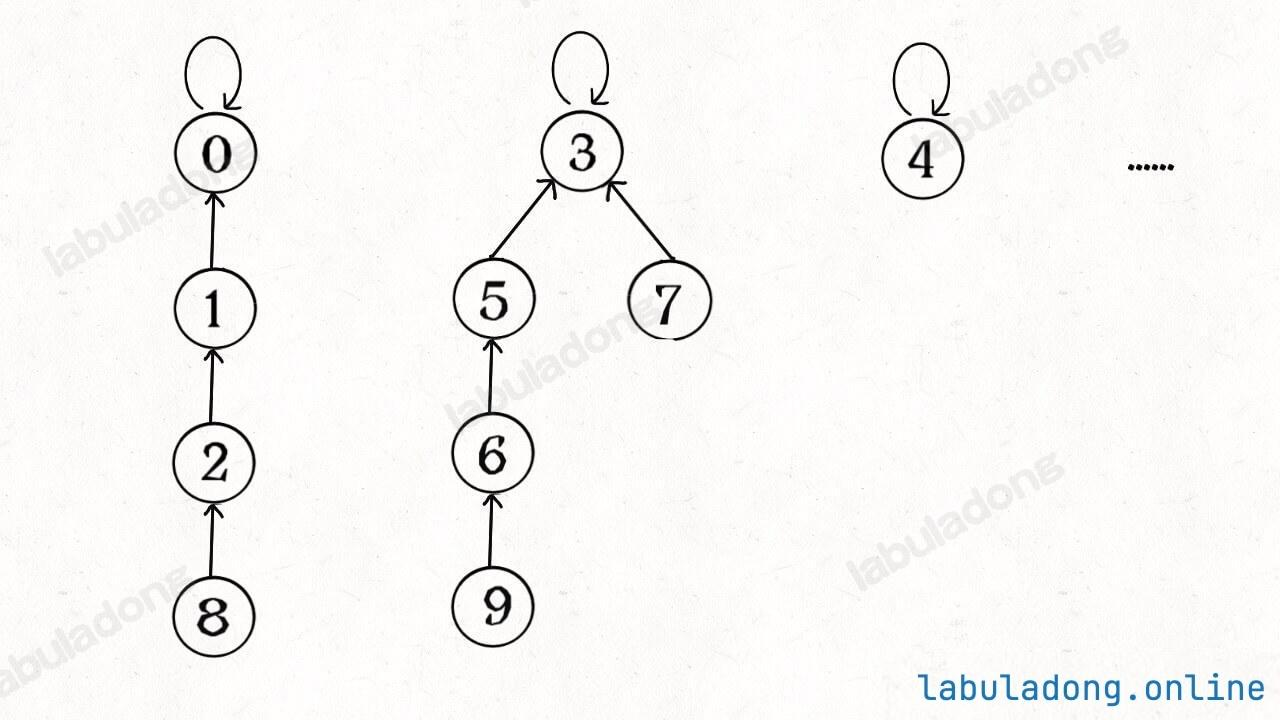### # 三、平衡性优化

``````class UF {
// 为了节约篇幅，省略上文给出的代码部分...

public void union(int p, int q) {
int rootP = find(p);
int rootQ = find(q);
if (rootP == rootQ)
return;
// 将两棵树合并为一棵
parent[rootP] = rootQ;
// parent[rootQ] = rootP 也可以
count--;
}
}
````````````class UF {
private int count;
private int[] parent;
// 新增一个数组记录树的“重量”
private int[] size;

public UF(int n) {
this.count = n;
parent = new int[n];
// 最初每棵树只有一个节点
// 重量应该初始化 1
size = new int[n];
for (int i = 0; i < n; i++) {
parent[i] = i;
size[i] = 1;
}
}
/* 其他函数 */
}
``````

``````class UF {
// 为了节约篇幅，省略上文给出的代码部分...

public void union(int p, int q) {
int rootP = find(p);
int rootQ = find(q);
if (rootP == rootQ)
return;

// 小树接到大树下面，较平衡
if (size[rootP] > size[rootQ]) {
parent[rootQ] = rootP;
size[rootP] += size[rootQ];
} else {
parent[rootP] = rootQ;
size[rootQ] += size[rootP];
}
count--;
}
}
``````

### # 四、路径压缩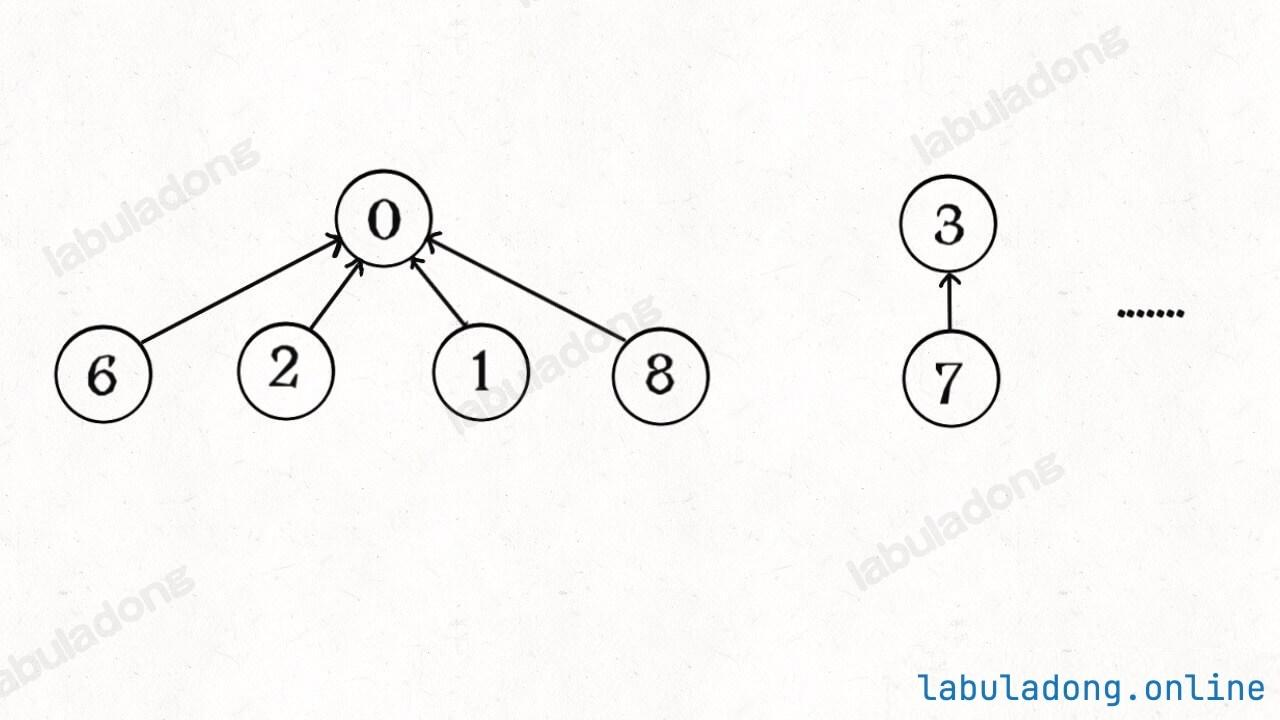``````class UF {
// 为了节约篇幅，省略上文给出的代码部分...

private int find(int x) {
while (parent[x] != x) {
// 这行代码进行路径压缩
parent[x] = parent[parent[x]];
x = parent[x];
}
return x;
}
}
``````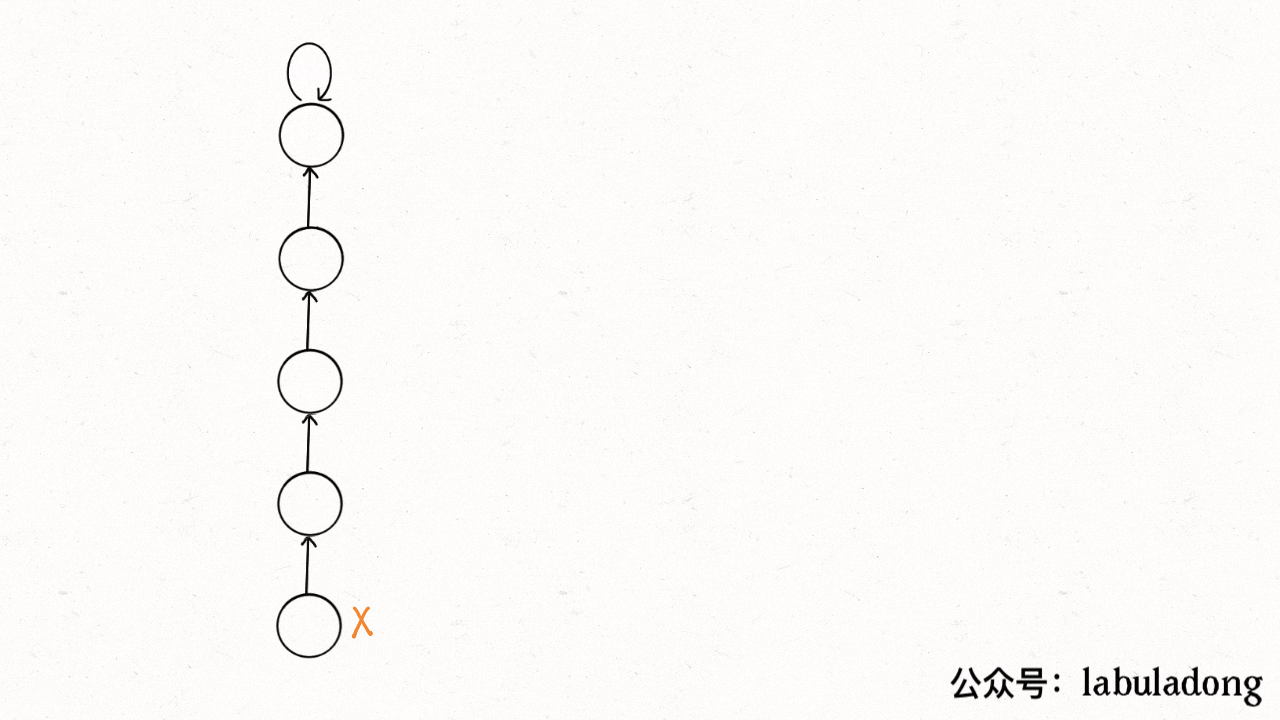``````class UF {
// 为了节约篇幅，省略上文给出的代码部分...

// 第二种路径压缩的 find 方法
public int find(int x) {
if (parent[x] != x) {
parent[x] = find(parent[x]);
}
return parent[x];
}
}
``````

``````// 这段迭代代码方便你理解递归代码所做的事情
public int find(int x) {
// 先找到根节点
int root = x;
while (parent[root] != root) {
root = parent[root];
}
// 然后把 x 到根节点之间的所有节点直接接到根节点下面
int old_parent = parent[x];
while (x != root) {
parent[x] = root;
x = old_parent;
old_parent = parent[old_parent];
}
return root;
}
``````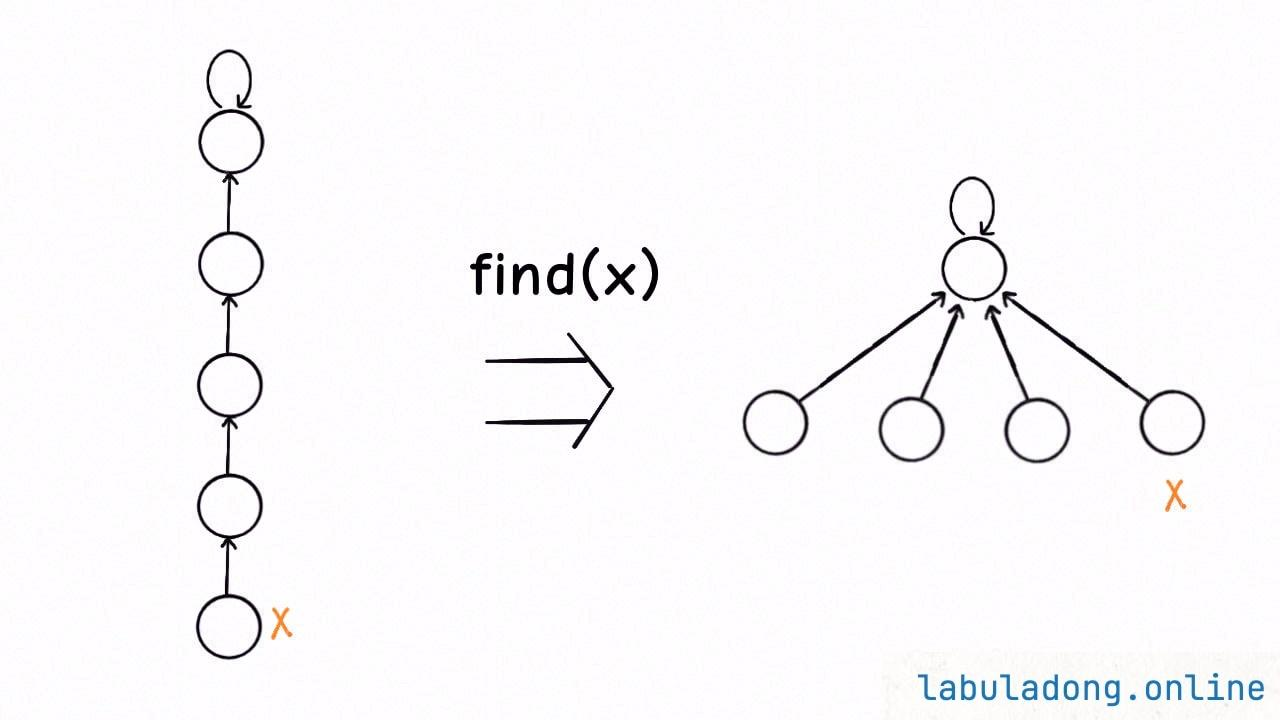``````class UF {
// 连通分量个数
private int count;
// 存储每个节点的父节点
private int[] parent;

// n 为图中节点的个数
public UF(int n) {
this.count = n;
parent = new int[n];
for (int i = 0; i < n; i++) {
parent[i] = i;
}
}

// 将节点 p 和节点 q 连通
public void union(int p, int q) {
int rootP = find(p);
int rootQ = find(q);

if (rootP == rootQ)
return;

parent[rootQ] = rootP;
// 两个连通分量合并成一个连通分量
count--;
}

// 判断节点 p 和节点 q 是否连通
public boolean connected(int p, int q) {
int rootP = find(p);
int rootQ = find(q);
return rootP == rootQ;
}

public int find(int x) {
if (parent[x] != x) {
parent[x] = find(parent[x]);
}
return parent[x];
}

// 返回图中的连通分量个数
public int count() {
return count;
}
}
``````

Union-Find 算法的复杂度可以这样分析：构造函数初始化数据结构需要 O(N) 的时间和空间复杂度；连通两个节点 `union`、判断两个节点的连通性 `connected`、计算连通分量 `count` 所需的时间复杂度均为 O(1)。

1、用 `parent` 数组记录每个节点的父节点，相当于指向父节点的指针，所以 `parent` 数组内实际存储着一个森林（若干棵多叉树）。

2、用 `size` 数组记录着每棵树的重量，目的是让 `union` 后树依然拥有平衡性，保证各个 API 时间复杂度为 O(logN)，而不会退化成链表影响操作效率。

3、在 `find` 函数中进行路径压缩，保证任意树的高度保持在常数，使得各个 API 时间复杂度为 O(1)。使用了路径压缩之后，可以不使用 `size` 数组的平衡优化。

### # 题目实践

``````int countComponents(int n, int[][] edges)
``````

``````public int countComponents(int n, int[][] edges) {
UF uf = new UF(n);
// 将每个节点进行连通
for (int[] e : edges) {
uf.union(e, e);
}
// 返回连通分量的个数
return uf.count();
}

class UF {
// 见上文
}
``````

``````void solve(char[][] board);
``````Note``````void solve(char[][] board) {
if (board.length == 0) return;

int m = board.length;
int n = board.length;
// 给 dummy 留一个额外位置
UF uf = new UF(m * n + 1);
int dummy = m * n;
// 将首列和末列的 O 与 dummy 连通
for (int i = 0; i < m; i++) {
if (board[i] == 'O')
uf.union(i * n, dummy);
if (board[i][n - 1] == 'O')
uf.union(i * n + n - 1, dummy);
}
// 将首行和末行的 O 与 dummy 连通
for (int j = 0; j < n; j++) {/**<extend up -150><div class="img-content"><img src="/algo/images/unionfind应用/3.jpg" class="myimage"/></div> */
if (board[j] == 'O')
uf.union(j, dummy);
if (board[m - 1][j] == 'O')
uf.union(n * (m - 1) + j, dummy);
}
// 方向数组 d 是上下左右搜索的常用手法
int[][] d = new int[][]{{1,0}, {0,1}, {0,-1}, {-1,0}};
for (int i = 1; i < m - 1; i++)
for (int j = 1; j < n - 1; j++)
if (board[i][j] == 'O')
// 将此 O 与上下左右的 O 连通
for (int k = 0; k < 4; k++) {
int x = i + d[k];
int y = j + d[k];
if (board[x][y] == 'O')
uf.union(x * n + y, i * n + j);
}
// 所有不和 dummy 连通的 O，都要被替换
for (int i = 1; i < m - 1; i++)
for (int j = 1; j < n - 1; j++)
if (!uf.connected(dummy, i * n + j))
board[i][j] = 'X';
}

class UF {
// 见上文
}
``````

``````boolean equationsPossible(String[] equations) {
// 26 个英文字母
UF uf = new UF(26);
// 先让相等的字母形成连通分量
for (String eq : equations) {
if (eq.charAt(1) == '=') {
char x = eq.charAt(0);
char y = eq.charAt(3);
uf.union(x - 'a', y - 'a');
}
}
// 检查不等关系是否打破相等关系的连通性
for (String eq : equations) {
if (eq.charAt(1) == '!') {
char x = eq.charAt(0);
char y = eq.charAt(3);
// 如果相等关系成立，就是逻辑冲突
if (uf.connected(x - 'a', y - 'a'))
return false;
}
}
return true;
}

class UF {
// 见上文
}
``````